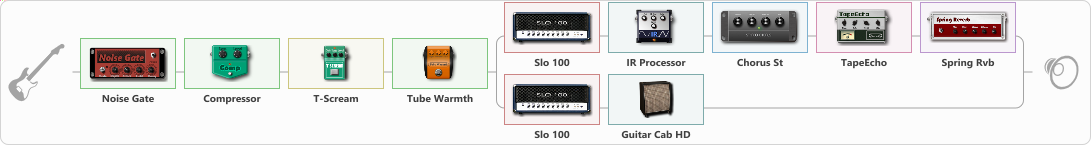# Soldano sound 2

Discussion in 'ToneLib-GFX presets' started by ktaxi, Sep 20, 2022.

1. Soldano sound 2

Preset name: The Soldano sound

Bridge pickup,i hope you like it!!!

Effects chain:Effect: "Noise Gate" (Dynamics / Filter), active - "yes"
{
"Mode" = Manual
"Depth" = 50
"Threshold" = 62
"Attack" = 0
"Hold" = 5
"Decay" = 250
}

Effect: "Compressor" (Dynamics / Filter), active - "yes"
{
"Sense" = 40
"Level" = 37
}

Effect: "T-Scream" (Overdrive / Distortion), active - "yes"
{
"Drive" = 22
"Tone" = 53
"Level" = 56
}

Effect: "Tube Warmth" (Dynamics / Filter), active - "yes"
{
"Input" = 62
"Warmth" = 60
"Level" = 66
}

Effect: "Splitter" (Dynamics / Filter)
{
"A-Bypass" = Off
"A-Pan" = -35
"A-Level" = 62
"B-Bypass" = Off
"B-Pan" = 35
"B-Level" = 65
"Width" = 25

'A' branch:
{

Effect: "Slo 100" (Amp simulators), active - "yes"
{
"Gain" = 60
"Bass" = 94
"Middle" = 94
"Treble" = 59
"Presence" = 52
"Master" = 90
"Level (dB)" = 2
}

Effect: "IR Processor" (Cabinets), active - "yes"
{
"IR" = Cab IR Sample4
"Low Cut (Hz)" = 0
"Hi Cut (kHz)" = 20.0
"Mix" = 100
"Level (dB)" = 0
}

Effect: "Chorus St" (Modulation / Sfx), active - "yes"
{
"Speed" = 1.9
"Depth" = 26
"Center" = 3.9
"Mix" = 27
}

Effect: "TapeEcho" (Delay), active - "yes"
{
"Time" = 419
"Feedback" = 22
"Tone" = 54
"LoDamp" = 19
"Mix" = 57
}

Effect: "Spring Rvb" (Reverberation), active - "yes"
{
"Time" = 3.8
"PreDelay" = 0
"LoDamp" = 16
"HiDamp" = 23
"Mix" = 27
}
}
'B' branch:
{

Effect: "Slo 100" (Amp simulators), active - "yes"
{
"Gain" = 57
"Bass" = 100
"Middle" = 100
"Treble" = 87
"Presence" = 55
"Master" = 90
"Level (dB)" = 2
}

Effect: "Guitar Cab HD" (Cabinets), active - "yes"
{
"Model" = Soldano Slant (4x12")
"Mic Model" = Dynamic SM57
"Mic Position" = Middle
"Mic Distance" = Middle
"Low Cut (Hz)" = 80
"Hi Cut (kHz)" = 20.0
"Mix" = 100
"Level (dB)" = 0
}
}
}

Note: You will need to download and install the ToneLib-GFX software to use the preset.

File size:
4.3 KB
Views:
2,230